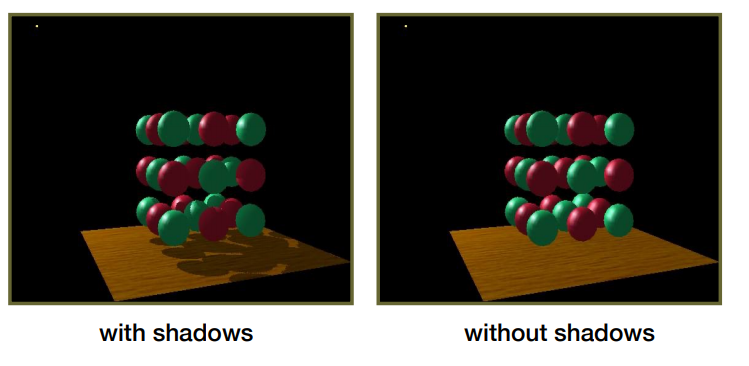1. 阴影生成 Pass：
• 额外设置一个摄像机在光源位置（Light Camera，光源摄像机），并且朝光照方向看去。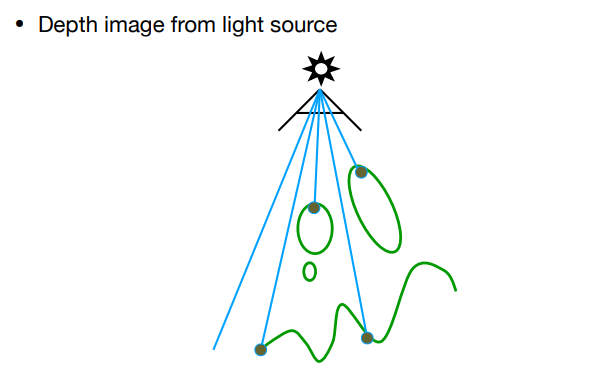// shadowVertex.glsl
// ...
void main(void) {
vNormal = aNormalPosition;
vTextureCoord = aTextureCoord;
gl_Position = uLightMVP * vec4(aVertexPosition, 1.0);
}

// shadowFragment.glsl
// ...
void main(){
gl_FragColor = pack(gl_FragCoord.z);
}1. 渲染 Pass：
• 主摄像机需要渲染屏幕每个像素时，该像素对应的世界坐标进行 Light Camera 的MVP变换后能得到在 Light Camera 屏幕空间中的对应位置 $$shadowCoord = (x',y',z')$$
• Shadow Map 里用$$(x',y')$$采样得到的遮挡深度 $$depth$$ 与深度值 $$z'$$ 做比较： 若 $$depth < z'$$（意味着该像素的光被遮挡），这时就可以对该像素降低可见度（Visibility）。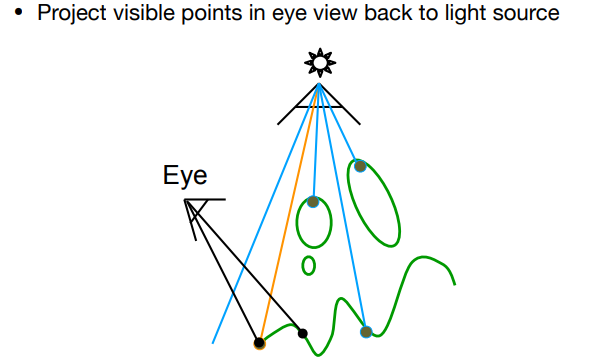// phongVertex.glsl
// ...
void main(void) {
vNormal = (uModelMatrix * vec4(aNormalPosition, 0.0)).xyz;
vTextureCoord = aTextureCoord;
vFragPos = (uModelMatrix * vec4(aVertexPosition, 1.0)).xyz;
vPositionFromLight = uLightMVP * vec4(aVertexPosition, 1.0);
gl_Position = uProjectionMatrix * uViewMatrix * uModelMatrix * vec4(aVertexPosition, 1.0);
}

// phongFragment.glsl
// ...
void main(){
// 归一化坐标
vec3 projCoords = vPositionFromLight.xyz / vPositionFromLight.w;
vec3 shadowCoord = projCoords * 0.5 + 0.5;
float visibility = 1.0;
visibility = 0.0;
}
// blinnPhong光照着色
vec3 color = blinnPhong();

gl_FragColor = vec4(color * visibility,1.0);
}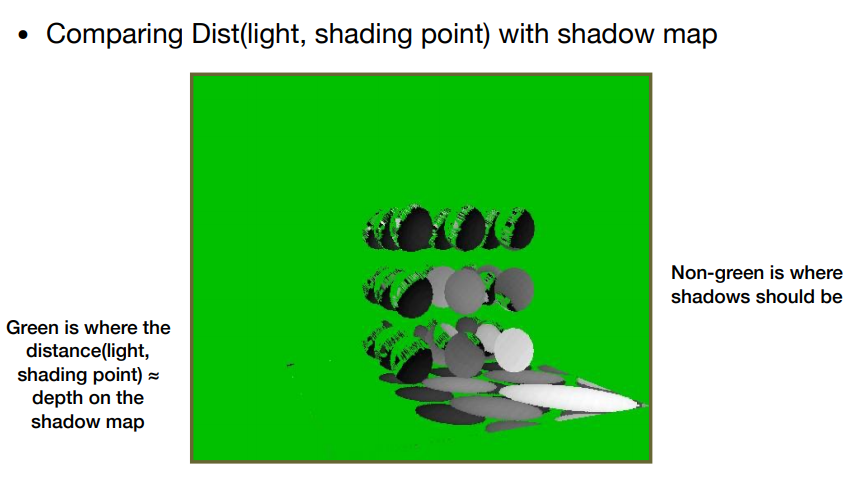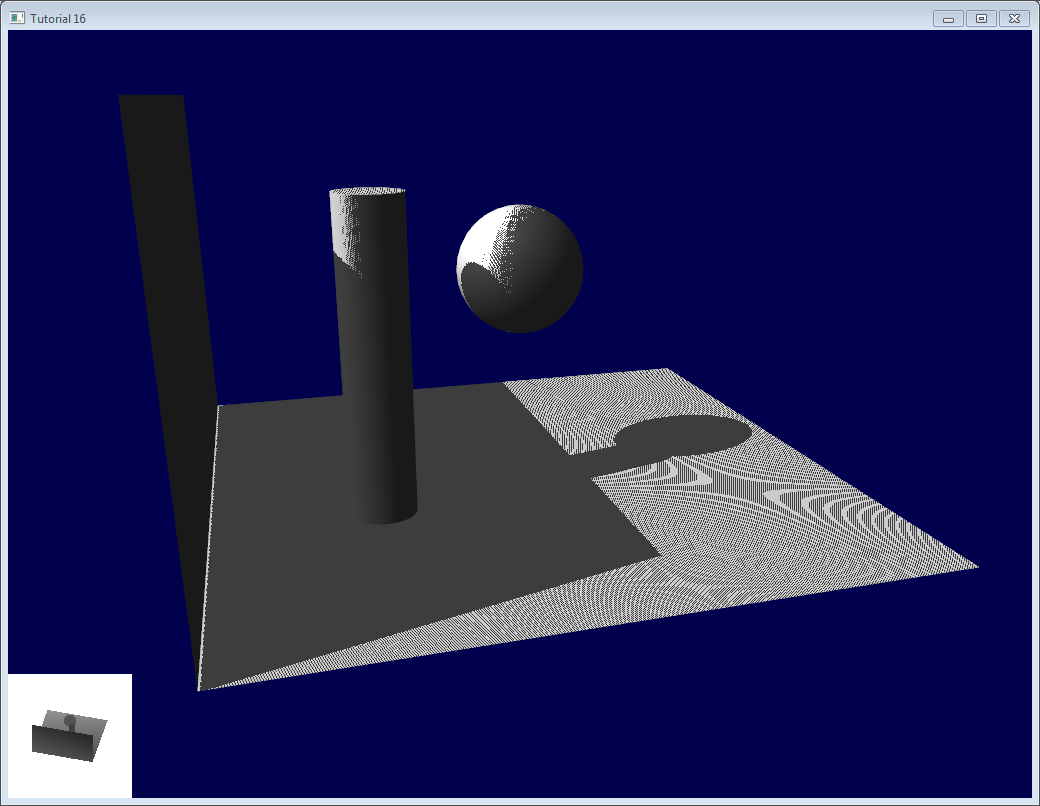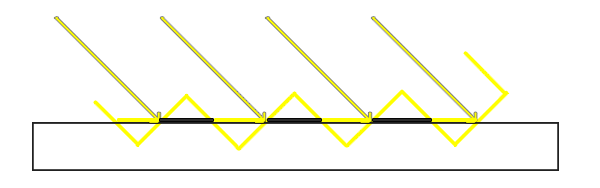• 直接给采样阴影深度加一个 偏移量 Bias（相当于把阴影深度往远处加，从而更不容易产生遮挡）。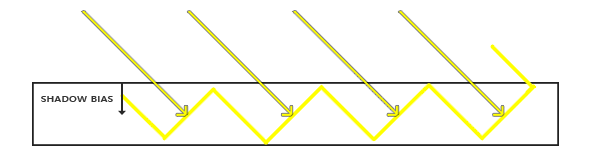// phongFragment.glsl
//...
void main(){
// 归一化坐标
vec3 projCoords = vPositionFromLight.xyz / vPositionFromLight.w;
vec3 shadowCoord = projCoords * 0.5 + 0.5;
const float BIAS = 0.005;
float visibility = 1.0;
visibility = 0.0;
}
// blinnPhong光照着色
vec3 color = blinnPhong();

gl_FragColor = vec4(color * visibility,1.0);
}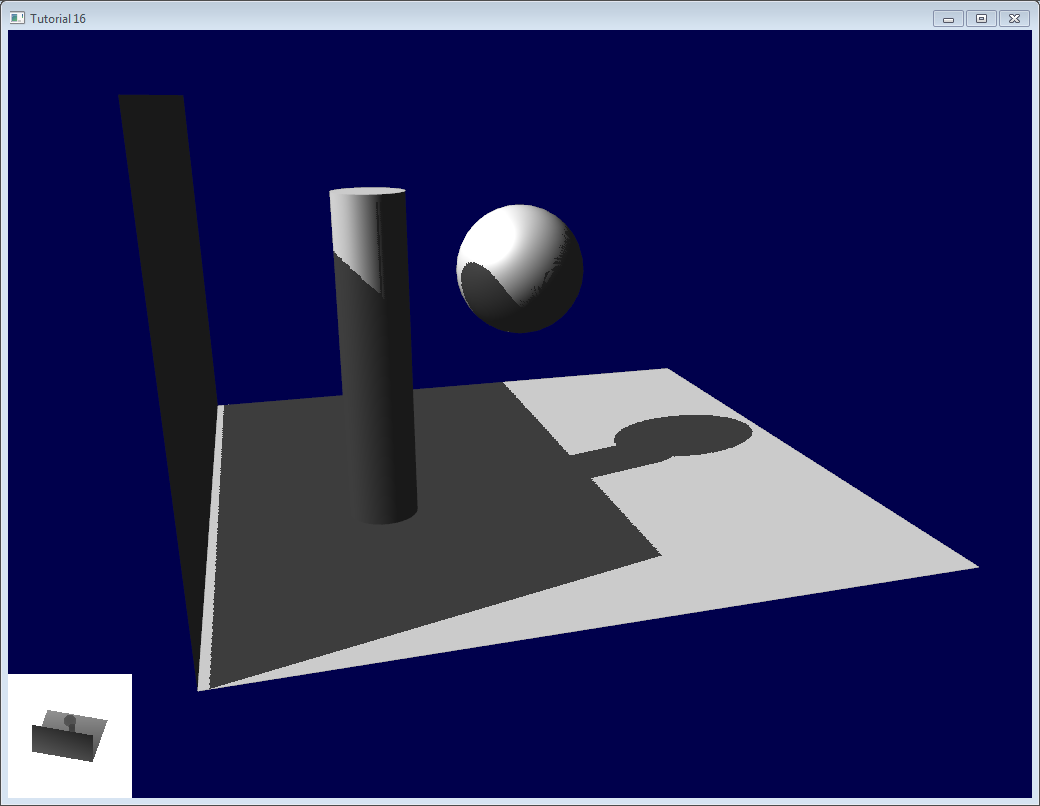## Peter Panning 问题 & 简单 Trick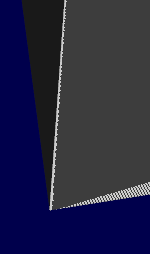• 避免使用单薄的几何体（例如薄墙、薄地面）；只要几何体厚度大于Bias，影子边界便会产生在几何体内部，从而不易看见影子与几何体的分离现象。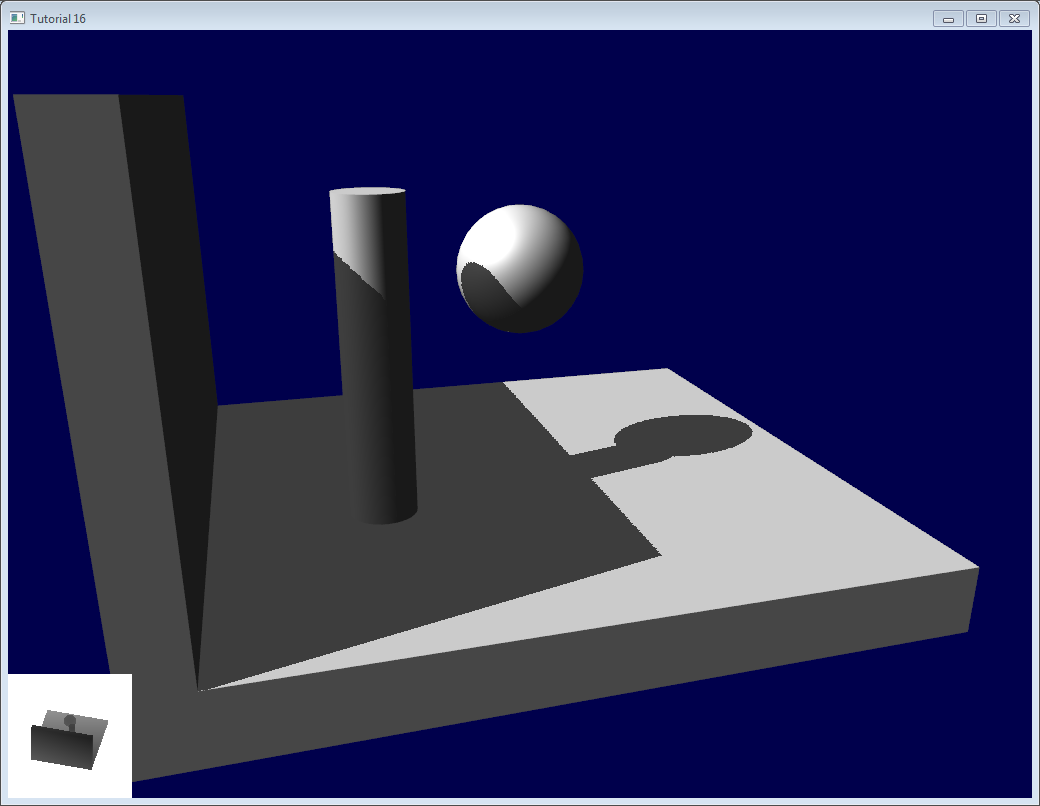• 不使用Bias

• 第一个Pass（Light Camera记录深度的那个）设置成仅渲染背面（正面剔除）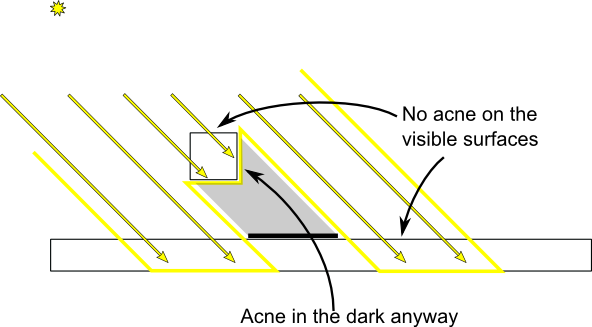## Slope Scale Based Depth Bias

Slope Scale Based Depth Bias ：为了尽可能减少由于 Bias 过大过小引起的问题，采取了根据平面倾角的一种自适应 Bias（例如：当光线与平面垂直时，Bias应该为0；当光线与平面的夹角越小，则Bias应越大）。

float bias = max(0.05 * (1.0 - dot(normal, lightDir)), 0.005);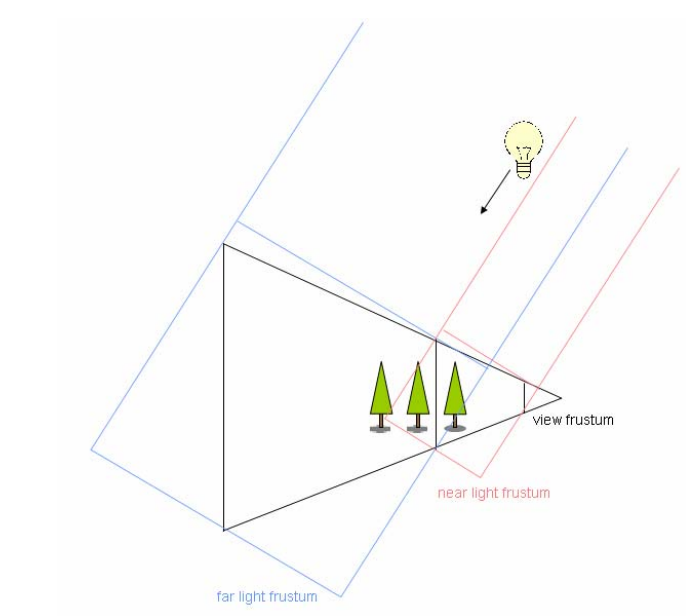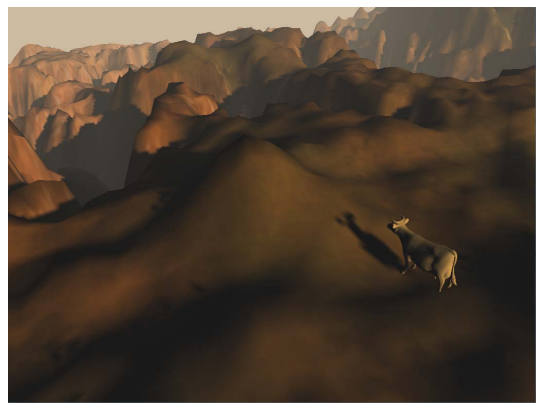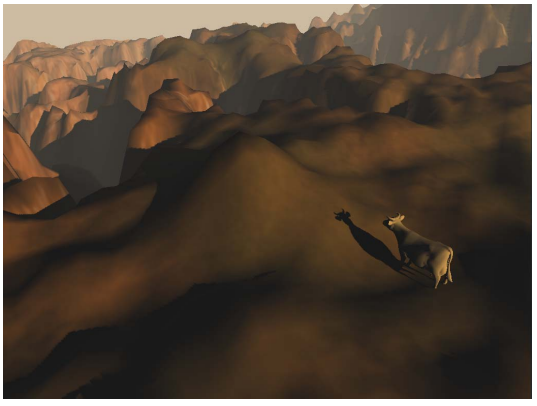## 视锥分割

• 通过自定义比例来分割

• 通过对数比例分割：这是较理想的比例公式，能够减少 Shadow Perspective Alias 问题的出现（下面是公式推理的内容）。

$$\frac{dp}{ds} = \frac{n\tan(\theta)dz}{z}/ ds$$

$$\varphi$$$$\theta$$ 为物体平面法线的角度参数，则 $$\tan(\theta)=\frac{\sin(\theta)}{\cos(\theta)}=\frac{\cos(\varphi)}{\cos(\theta)}$$

$$n$$ 为主摄像机近平面距离，$$f$$ 为主摄像机远平面距离。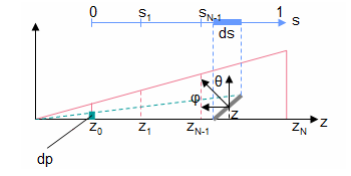$$\frac{dp}{ds} = C_0$$

$$\frac{1}{z}\frac{dz}{ds} = C$$

$$z=n \cdot \left(\frac{f}{n}\right)^{s}$$

$$z_i=n \cdot (\frac{f}{n})^{\frac{i}{N}},i=1,...,N$$

$$z_{i}=\operatorname{lerp}\left(n *(\frac{f}{n})^{\frac{i}{N}}, n+\frac{i*(f-n)}{N}, \lambda\right), i=1, \ldots, N \ , \lambda \in [0,1]$$

$$\lambda$$ 是一个 $$[0,1]$$ 区间的控制参数，用以在对数和线性之间进行插值

## 计算包围盒

• 使用最紧凑的方形包围盒：分割后的视锥体的8个顶点在世界空间上做的View变换后，取它们最大最小x值y值z值来作为 Light Camera 的远近平面参数设置。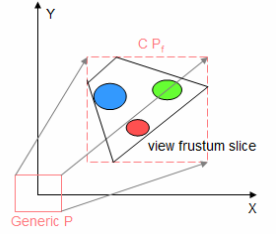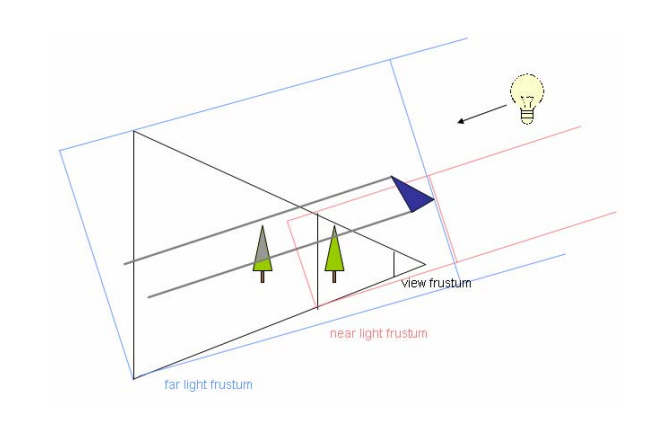## 层级选择

• 直接通过视锥分割的z值范围来判断所在是哪个层级

• 通过各层 Light Camera 的 View 变换和 Projection 变换，得到点在该层 Shadow Map 的 UV 坐标，当 UV 坐标在 [0,1] 范围内时则说明在该层级内

# Percentage Closer Filtering（PCF）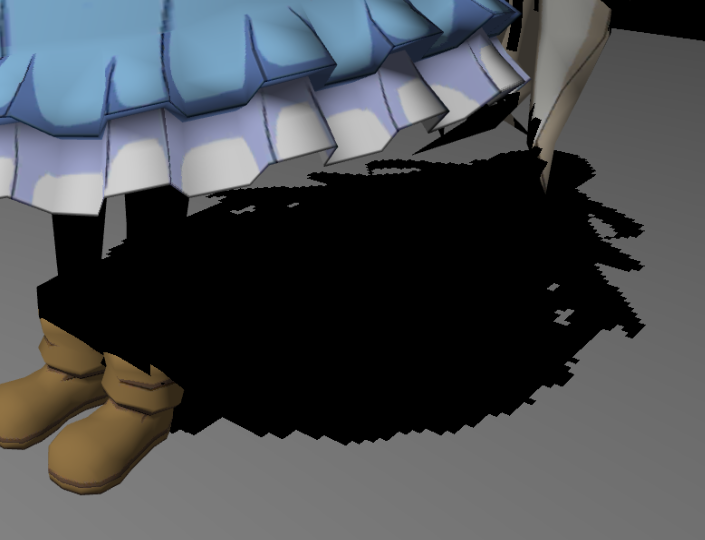Percentage Closer Filtering（PCF）正是解决阴影锯齿的方案，它的核心想法是计算阴影时不是考虑单个采样点，而是在一定范围内进行多重采样，这样可以让阴影的边缘不那么锯齿，因为 Visibility 不再是非0即1，而是带有渐变的取值。

## 分布采样函数

vec2 disk[NUM_SAMPLES]; // 经过分布采样函数运算后得到NUM_SAMPLES个采样坐标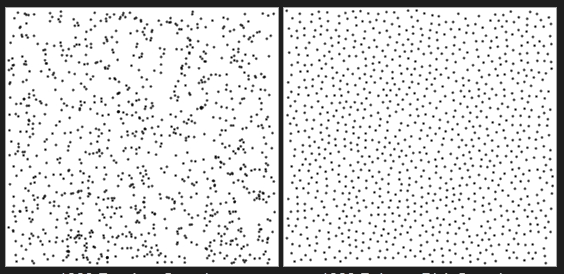// 均匀圆盘分布
void uniformDiskSamples( const in vec2 randomSeed ) {
// 随机种子
float randNum = rand_2to1(randomSeed);
// 随机取一个角度
float sampleX = rand_1to1( randNum ) ;
float angle = sampleX * PI2;
// 随机取一个半径
float sampleY = rand_1to1( sampleX ) ;
for( int i = 0; i < NUM_SAMPLES; i ++ ) {
// 继续随机取一个半径
sampleX = rand_1to1( sampleY ) ;
// 继续随机取一个角度
sampleY = rand_1to1( sampleX ) ;
angle = sampleX * PI2;
}
}

// 泊松圆盘分布
void poissonDiskSamples( const in vec2 randomSeed ) {
// 初始弧度
float angle = rand_2to1( randomSeed ) * PI2;
// 初始半径
float INV_NUM_SAMPLES = 1.0 / float( NUM_SAMPLES );
// 一步的弧度
float ANGLE_STEP = 3.883222077450933;// (sqrt(5)-1)/2 *2PI
// 一步的半径

for( int i = 0; i < NUM_SAMPLES; i ++ ) {
disk[i] = vec2(cos(angle),sin(angle)) * pow( radius, 0.75 );
angle += ANGLE_STEP;
}
}


## PCF 算法过程

Percentage Closer Filtering（PCF） 的算法过程：

1. 计算 Visibility 时，原本对 Shadow Map 的一次坐标采样换成对周围一定范围内若干个坐标进行采样。
2. 各个采样结果同样用来与 $$z'$$ 做比较，最后取比较结果的平均作为 Visibility。
float visibility_PCF(sampler2D shadowMap, vec4 coords) {
const float bias = 0.005;
float sum = 0.0;
// 初始化泊松分布
poissonDiskSamples(coords.xy);
// 采样
for(int i = 0;i<NUM_SAMPLES;++i){
sum += ((depthInShadowmap + bias)< coords.z?0.0:1.0);
}
// 返还平均采样结果
return sum/float(NUM_SAMPLES);
}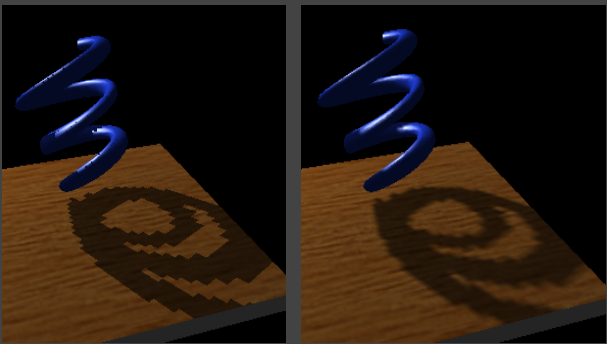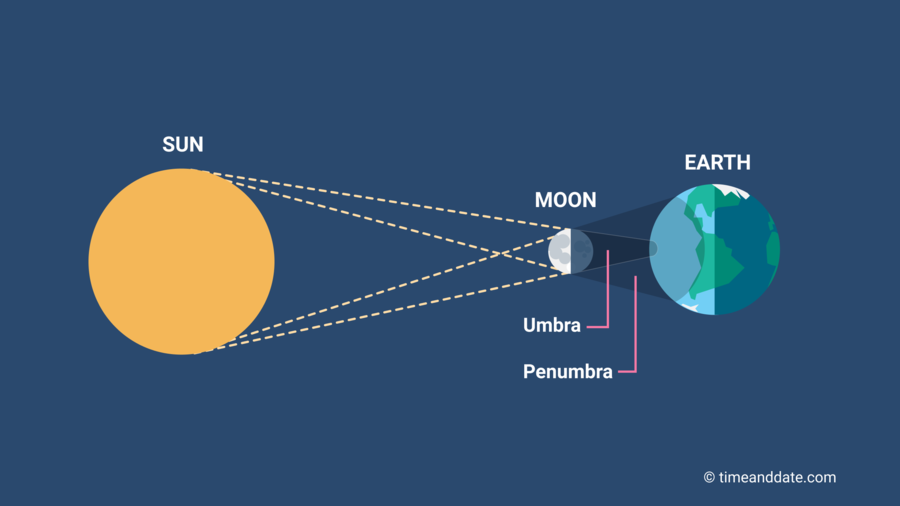## Penumbra Size

$$w_{\text {Penumbra }}=\left(d_{\text {Receiver }}-d_{\text {Blocker }}\right) \cdot w_{\text {Light }} / d_{\text {Blocker }}$$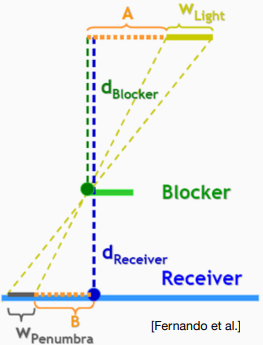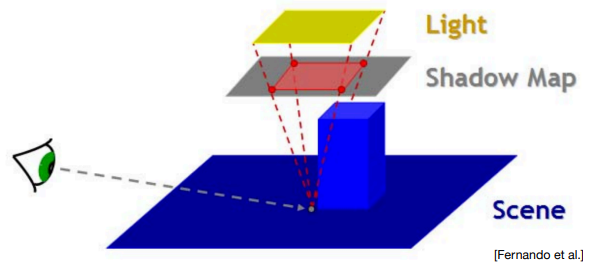$$SampleSize=w_{Light}\cdot z_{Receiver} \cdot c$$

float findBlocker( sampler2D shadowMap, vec2 uv, float zReceiver ) {  float dBlocker = zReceiver * 0.01;  const float wLight = 0.006;  const float c = 100.0;	  float sampleSize = wLight * zReceiver * c;  float sum = 0.01;	// 取0.01一是为了避免出现0除问题，二是当多重采样没有贡献时的dBlocker/sum将等于zReceiver  for(int i = 0;i<BLOCKER_SEARCH_NUM_SAMPLES;++i){    float depthInShadowmap = unpack(texture2D(shadowMap,uv+disk[i]*sampleSize).rgba);    if(depthInShadowmap < zReceiver){      dBlocker += depthInShadowmap;      sum += 1.0;    }  }  return dBlocker/float(sum);}


## PCSS 算法过程

1. Blocker Search：通过多重采样，计算出平均遮挡深度 $$d_{Blocker}$$

2. Penumbra Size：计算圆盘半径大小 $$w_{\text {Penumbra }}=\left(d_{\text {Receiver }}-d_{\text {Blocker }}\right) \cdot w_{\text {Light }} / d_{\text {Blocker }}$$

3. Filtering：通过多重采样，计算出平均 Visibility（实际上就是调用PCF算法）

float visibility_PCSS(sampler2D shadowMap, vec4 coords){
poissonDiskSamples(coords.xy);
// STEP 1: avgblocker depth
// STEP 2: penumbra size
const float wLight = 0.006;
float penumbra = (coords.z-dBlocker)/dBlocker * wLight;
// STEP 3: filtering
const float bias = 0.005;
float sum = 0.0;
for(int i = 0;i<PCF_NUM_SAMPLES;++i){
sum += ((depthInShadowmap + bias)< coords.z?0.0:1.0);
}
return sum/float(PCF_NUM_SAMPLES);
}


PCF算法效果图：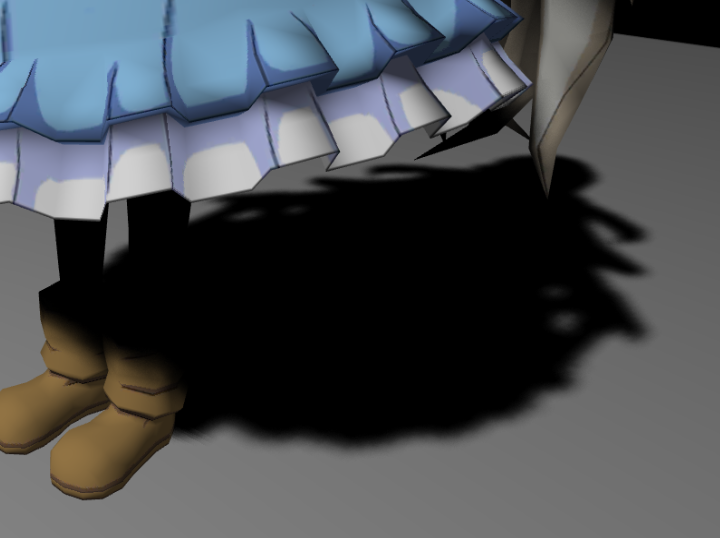PCSS算法效果图：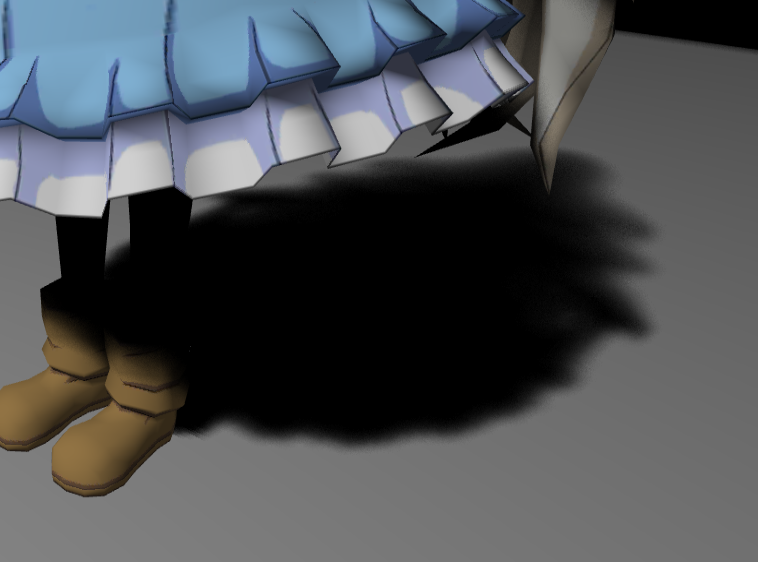PCSS、PCF 的算法都需要多重采样，尤其 PCSS 需要两个多重采样（第一步的Blocker Search和第三步的PCF），这使得算法速度较慢。

$$PDF(x) = \frac{1}{\sqrt{2 \pi} \sigma} \exp \left(-\frac{(x-\mu)^{2}}{2 \sigma^{2}}\right)$$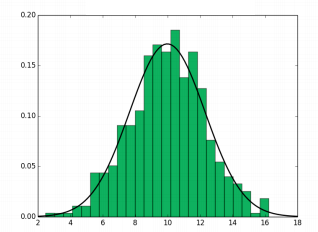$$CDF(x) = \int^x_{-\infin} PDF(t) \mathrm{d}t$$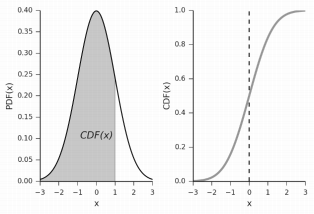VSSM效果图：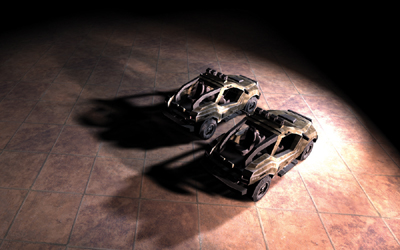## 计算平均值 & 方差

• 硬件 Mipmap：当 Shadow Map 更新时，需要重新生成 Mipmap，不过GPU硬件实现的 Mipmap 算法非常快的开销非常小；查询某段方形范围时，需要根据方形中心所在的位置（相对于周围四个纹素的坐标）、上下层级做三线性插值（Trillinear interpolation），得到的结果即是近似的均值（期望值）。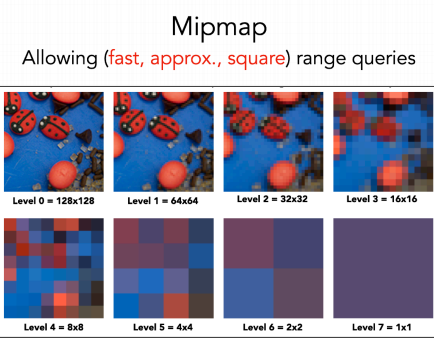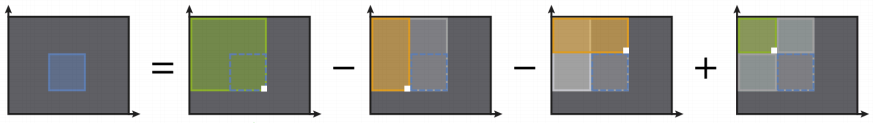1. 平均值 $$E(X)$$

2. 方差 $$Var(X)=E(X^2)-E^2(X)$$

$$E(X^2)$$ 即 ShadowMap 每个纹素再求个平方后作为额外的ShadowMap，然后再生成 Mipmap 或 SAT。

## 计算累计分布函数（CDF）$$P(x>t) \approx \frac{\sigma^{2}}{\sigma^{2}+(t-\mu)^{2}}$$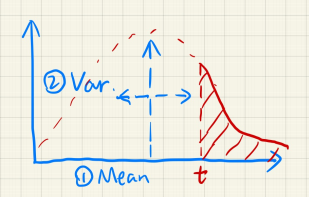## 加速 Blocker Search 算法

PCSS 算法中的 Blocker Search 步骤：在一定范围内多重采样，每次采样得到的深度若小于 $$d_{Receiver}$$ 则认为遇到遮挡物并算入平均遮挡深度的贡献，这样多重采样之后得到的平均遮挡深度 $$z_{occ}$$ 就作为 $$d_{Blocker}$$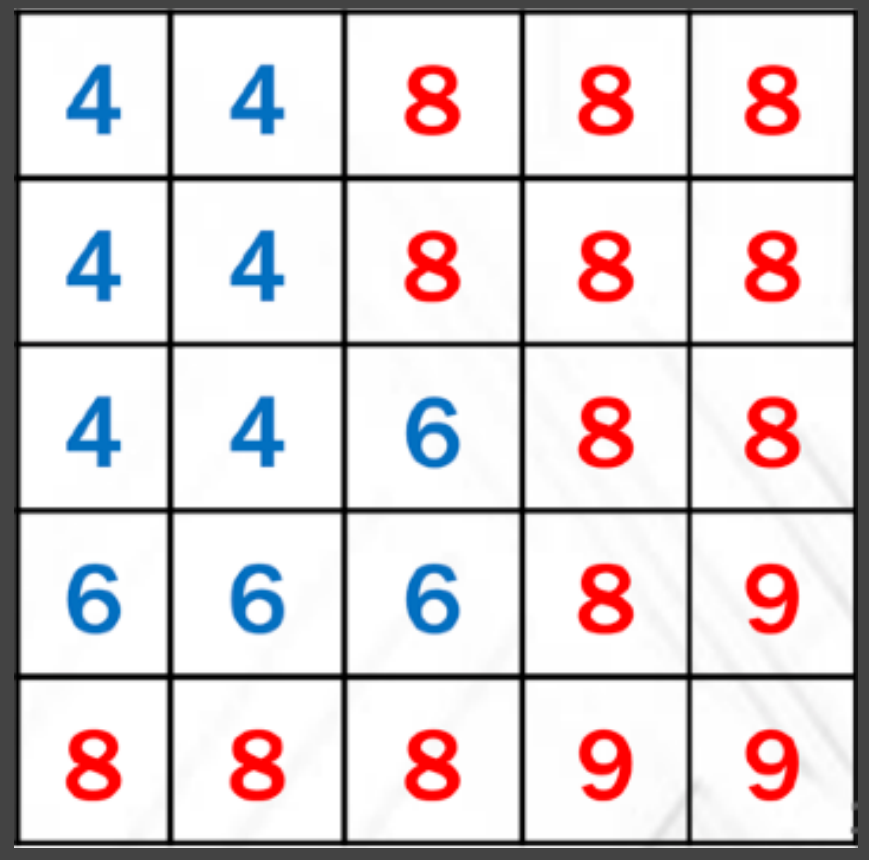$$\frac{N_{1}}{N} z_{\text {unocc }}+\frac{N_{2}}{N} z_{o c c}=z_{A v g}$$

• $$\frac{N_1}{N} = P(x>d_{Receiver})$$$$\frac{N_2}{N} = 1-P(x>d_{Receiver})$$ ；这个假设基于认为深度分布为正态分布，通过切克比夫不等式获得近似解（即上面两节的内容）。

• $$z_{unocc} = d_{Receiver}$$ ；这个假设基于认为绝大部分没被遮挡的情况都属于同一个深度（相当于在同一个垂直于光方向的平面），即可认为均为深度 $$d_{Receiver}$$

$$d_{Blocker} = z_{occ} = \frac{N\cdot z_{Avg} - N_1 \cdot z_{unocc}}{N_2} = \frac{E(x)-P(x>d_{Receiver})\cdot d_{Receiver}}{1-P(x>d_{Receiver})}$$

## 加速 PCF 算法

PCF 算法中的多重采样：每次采样得到的遮挡物深度用来与 $$z'$$ 做大小比较（小于 $$z'$$ 则视为被遮挡，大于 $$z'$$ 则视为全亮），最后取比较结果的平均作为 Visibility。

• $$不被遮挡的概率 = P(x > z')$$ ；这个假设基于认为深度分布为正态分布，通过切克比夫不等式获得近似解。

$$Visibility = P(x>z') \cdot 1 + (1-P(x>z')) \cdot 0 = P(x>z')$$

## VSSM 的缺陷

VSSM 的主要缺陷表现：

• 并不是任何深度的分布都是符合正态分布模型的，例如对于图右的简单几何体反而用正态分布表示会很不适合。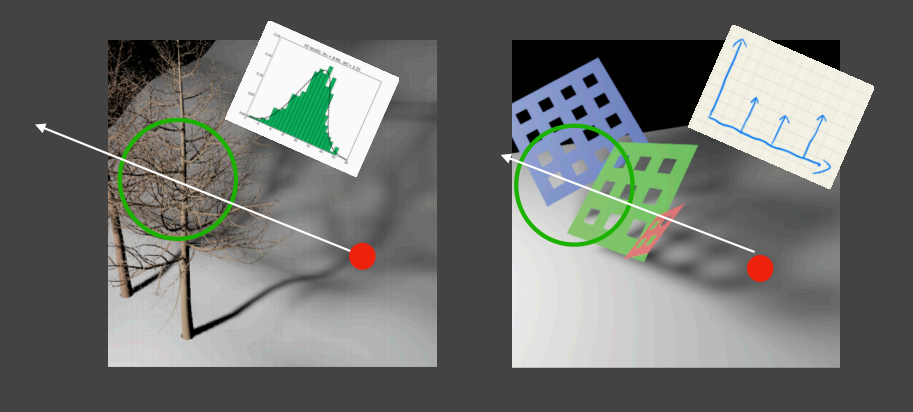• 漏光（Light Leaking）现象，在一些应当被阴影完全遮蔽的内部有可能仍产生亮度。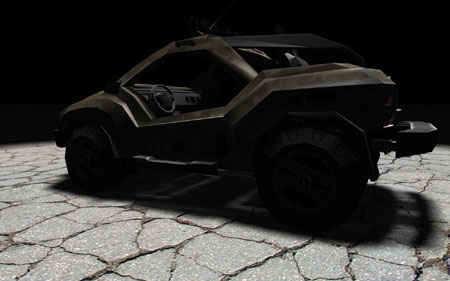• 在加速 Blocker Search 算法中的假设 $$z_{unocc} = d_{Receiver}$$ 基于认为绝大部分没被遮挡的情况都属于同一个深度，但实际上有些不被遮挡的地方深度并不等于 $$d_{Reveiver}$$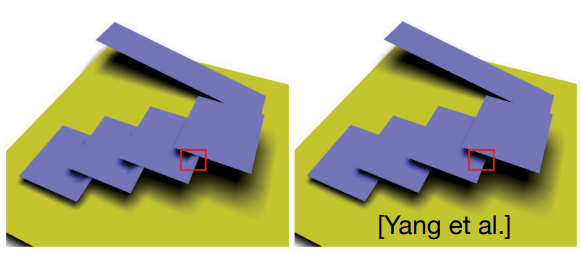Moment Shadow Mapping 正是为了解决 VSSM 缺陷的一种算法，它主要想法是：使用高阶的矩去描述一个分布的 CDF。这样就能通过记录 m 阶的矩，就能复原成足够接近实际 CDF 函数的效果，从而能适应不同的深度分布模型（有些地方可能接近正态分布，有些地方可能奇奇怪怪的分布）。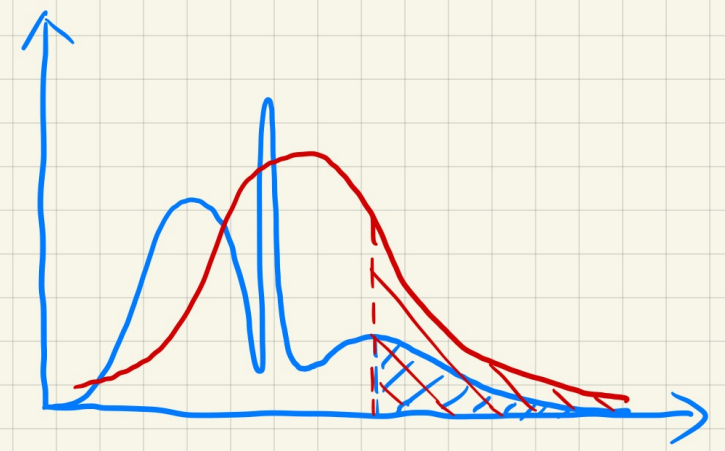Moment Shadow Mapping将使用最简单的形式来标识矩：$$z,z^2,z^3,z^4,...$$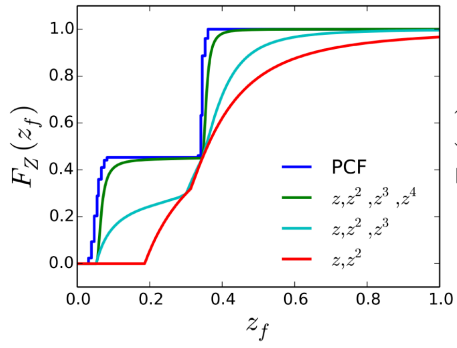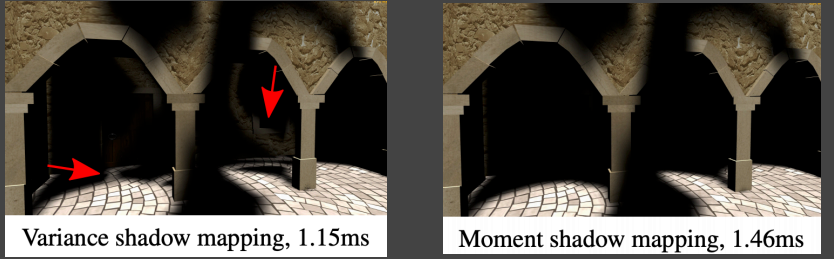SDF 相关可以看几何（Geometry）部分，这里假定已经对场景生成了 SDF 信息。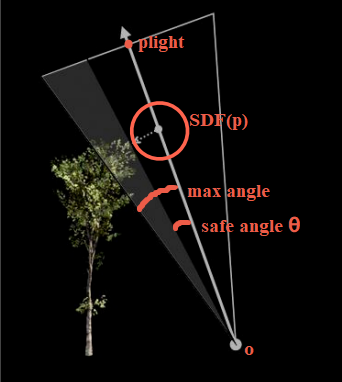• SDF 需要预计算，这就意味着场景物体需要是静态的，当然也可以使用一些算法使能和动态物体相结合，尽量减少重新生成SDF的成本。
• SDF 需要较大的存储空间（一般采用三维数组表示空间各个网格的SDF值，但是可以使用八叉树等空间数据结构或者其它方法做进一步优化）。

## 计算安全角度

$$\theta_i = \arcsin \frac{\operatorname{SDF}(p_i)}{p_i-o}$$

$$\theta_i = \min \left\{\frac{k \cdot \operatorname{SDF}(p_i)}{p_i-o}, 1.0\right\}$$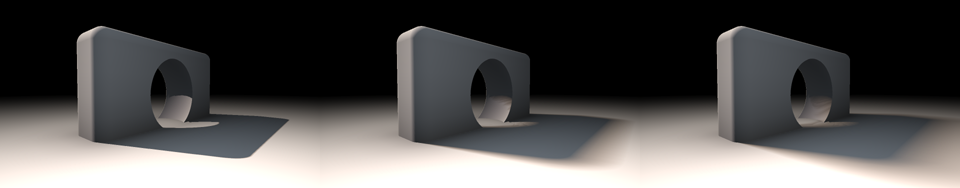## Distance Field Soft Shadows 算法过程

1. $$o$$ 点（shading point）设为第一个步进点，即 $$p_0 = o$$

2. 每次算出下一个步进点 $$p_{i+1} = p_{i} + l \cdot SDF(p_{i})$$ 并记录安全角度 $$\theta_i = \min \left\{\frac{k \cdot \operatorname{SDF}(p_i)}{p_i-o}, 1.0\right\}$$

3. 重复 "步骤2"，直到满足 $$l \cdot (p_{i+1}-p_{light}) < 0$$ （即意味着已经步进到光源点背面了）

4. 取所有次步进的最小安全角度 $$\theta = min\{\theta_i\}$$ ，则可见度则为 $$Visibility = \frac{\theta}{c}$$ （其中 $$c$$ 为点 $$o$$ 与光源面连接的最大角度）# 参考

posted @ 2021-08-28 22:08  KillerAery  阅读(2083)  评论(0编辑  收藏  举报